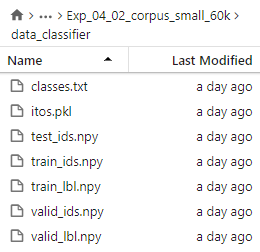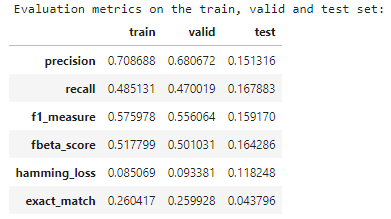# How to reliably use get_preds on the test set for ULMFiT?

When we process the `test_df` using `TextClasDataBunch`, the test_lbl.npy is not saved, i.e. the labels are saved only for the train and valid set, see:When we then call: `y_pred, y_true = classifier_learner.get_preds(ds_type = DatasetType.Test, with_loss=False)`, how this function can get the true labels? I think there might be a bug here, cause in the end, I get horrible results, especially on the test set (even though my data is quite clean atm):What is the correct way of getting predictions on the test set? I was thinking about manually ingesting my labels from `test_df`, but I am concerned about the order. I share my full notebook below.

The test set in fastai is unlabelled, it’s there to quickly get the predictions on a lot of unlabelled data. If you want to validate on a second set, you should create a second data object, as documented here.

thank you. How can I apply this to `from_df`? the example is for folders.

like this: `data_classifier.add_test(items = test_df)`?

"if you want to use a `test` dataset with labels, you probably need to use it as a validation set" --> but then doesn’t it defeat the purpose of the test set? cause then the test set would “leak” into the validation set.

I don’t understand, you don’t want to use `add_test` since you have labels.
In fastai:

• validation set = set with labels to check the performance
• test set = set without labels to get predictions on unlabelled data (like the test set in a kaggle competition)

If you want to validate on a set different from the valdation set, and create a second data object for it, it won’t ‘leak’ with the validation set you had before.

thank you. What does the function `learner.get_preds(DatasetType.Test)` return? It should return predictions and true labels. What does it return as true values then, if no labels are saved for the test set? Does it return correct labels of the test set?

It returns the predictions and an array of zeros (in 1.0.40) of the same size.

thx! Just now I used `learner.get_preds(DatasetType.Test, ordered=True)` and passed my own y_true array and it now works as it should.

Hi,

I am following up on this topic. When I used the method learner.get_preds(DatasetType.Test, ordered=True), I got really bad AUC score, although if I passed that “Test” set as the validation set, then I got really high AUC score, so somethings must be wrong.

One potential solution is to pass int Test set as validation set, but then I would have to train the model every time to get the predictions from learn.get_preds(ds_type=DatasetType.Valid). What if I have a complete new dataset and want to get the predictions from the trained learner?

`learner.get_preds(DatasetType.Test, ordered=True)` is exactly the command to get the predictions on a trained learner. I don’t know how you can have different predictions for this or when you put it as the validation set.